# Statistics - Standard Error (SE)

Standard Error is a measure of precision for a statistic (slope, intercept or custom calculations).

Standard error is an estimate of amount of sampling error as we typically don’t know the population parameters and that we are using a sample.

The standard error of an estimator reflects how it varies under repeated sampling (ie repeated training set).

Standard Error can be seen as the standard deviation of the error distribution. It determines the spread of the x's around the mean.

From two data set from the same population, we can get for the slope 0,5 or - 0.1 for instance. Standard Error permits to say how close is a coefficient to 0.

How much sampling error are we going to get, just due to chance. The standard error defines what you will just get due to chance.

Standard Error is the average amount of sampling error.

## Formulas

### Influence

Standard error and therefore sampling error are determined by:

### Bias

Standard error is biased by N as you can see in the formulas. Which means if N is increased:

• standard error will go down.
• the t-value will go way up
• and the p-value will go down.

Recommended Pages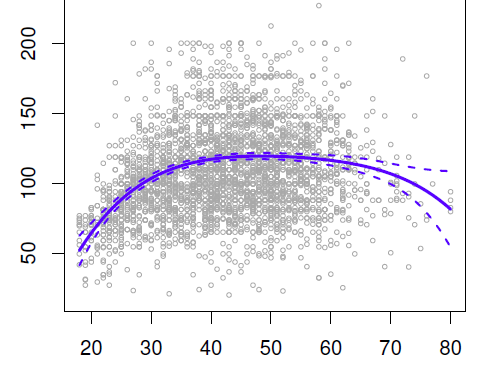Data Mining - (Global) Polynomial Regression (Degree)

polynomials regression Although polynomials are easy to think of, splines are much better behaved and more local. With polynomial regression, you create new variables that are just transformations...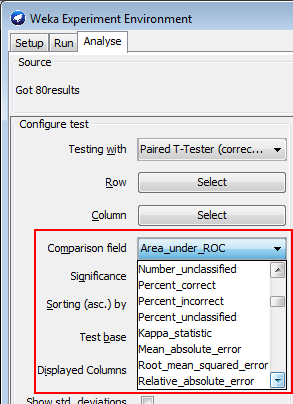Data Mining - (Parameters | Model) (Accuracy | Precision | Fit | Performance) Metrics

Accuracy is a evaluation metrics on how a model perform. rare event detection Hypothesis testing: t-statistic and p-value. The p value and t statistic measure how strong is the...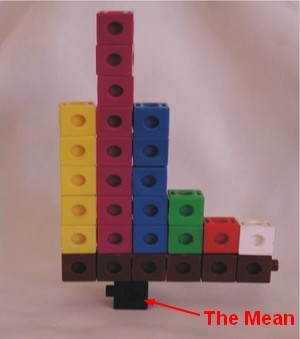Distribution - (Mean|Average) (M| | )

The average is a measure of center that statisticians call the mean. To calculate the mean, you add all numbers and divide the total by the number of numbers (N). The mean is not resistant. The...Machine Learning - (Overfitting|Overtraining|Robust|Generalization) (Underfitting)

A learning algorithm is said to overfit if it is: more accurate in fitting known data (ie training data) (hindsight) but less accurate in predicting new data (ie test data) (foresight) Ie the model...R - Bootstrap

in R. Bootstrap lets you get a look at the sampling distribution of statistics, for which it's really hard to develop theoretical versions. Bootstrap gives us a really easy way of doing statistics when...Statistics - (Student's) t-test (Mean Comparison)

The t-test is a test that compares means. NHST can be conducted yielding to a p-value Effect Size can be calculated like in multiple regression. Confidence Interval around the mean can also be...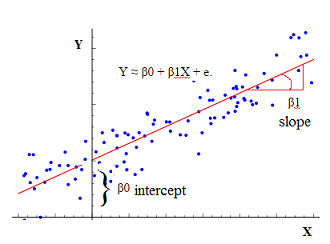Statistics - (Univariate|Simple|Basic) Linear Regression

A Simple Linear regression is a linear regression with only one predictor variable (X). Correlation demonstrates the relationship between two variables whereas a simple regression provides an equation...Statistics - (dependent|paired sample) t-test

A dependent t-test is appropriate when: we have the same people measured twice. the same subject are been compared (ex: Pre/Post Design) or two samples are matched at the level of individual subjects...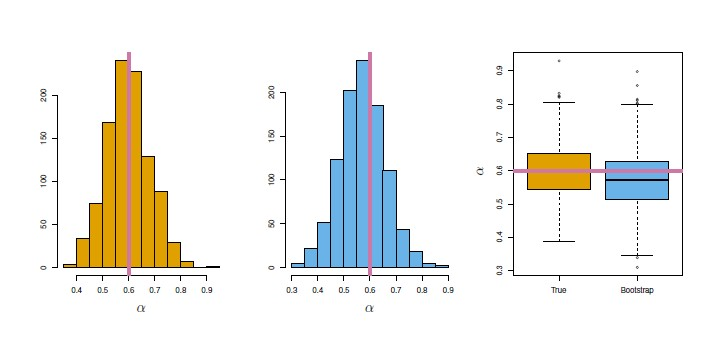Statistics - Bootstrap Resampling

Bootstrap is a powerful resampling method for assessing uncertainty in estimates and is particularly good for getting their: standard errors and confidence limits. Why is the bootstrap useful? The...Statistics - Confidence Interval

The definition of a confidence interval says that under repeated experiments 95% of the time this confidence interval will contain the true statistic (mean, ...). A 95% confidence interval is defined...